# Intermediate Geometry : How to find the area of a parallelogram

## Example Questions

1 2 3 4 6 Next →

### Example Question #51 : How To Find The Area Of A Parallelogram

Find the area of the parallelogram.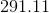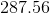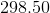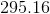Explanation:Recall how to find the area of a parallelogram: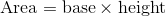From the given parallelogram, we will need to use the Pythagorean Theorem to find the length of the height of the parallelogram.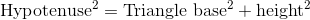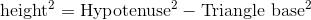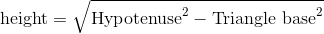Plug in the given values to find the length of the height.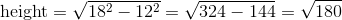Now, use the height to find the area of the parallelogram.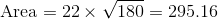Remember to round toplaces after the decimal.

### Example Question #52 : How To Find The Area Of A Parallelogram

Find the area of the parallelogram.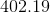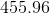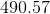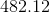Explanation:Recall how to find the area of a parallelogram:From the given parallelogram, we will need to use the Pythagorean Theorem to find the length of the height of the parallelogram.Plug in the given values to find the length of the height.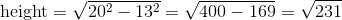Now, use the height to find the area of the parallelogram.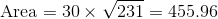Remember to round toplaces after the decimal.

### Example Question #53 : How To Find The Area Of A Parallelogram

Find the area of the parallelogram.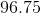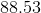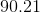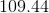Explanation:Recall how to find the area of a parallelogram:From the given parallelogram, we will need to use the Pythagorean Theorem to find the length of the height of the parallelogram.Plug in the given values to find the length of the height.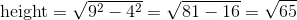Now, use the height to find the area of the parallelogram.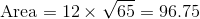Remember to round toplaces after the decimal.

### Example Question #54 : How To Find The Area Of A Parallelogram

Find the area of the parallelogram.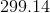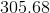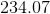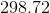Explanation:Recall how to find the area of a parallelogram:From the given parallelogram, we will need to use the Pythagorean Theorem to find the length of the height of the parallelogram.Plug in the given values to find the length of the height.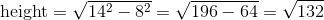Now, use the height to find the area of the parallelogram.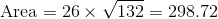Remember to round toplaces after the decimal.

### Example Question #55 : How To Find The Area Of A Parallelogram

84

100

112

56

42

84

Explanation:

The area of a parallelogram can be found using the following equation: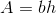In this problem, the height is 6 (not 8). Substitute the known variables and solve.### Example Question #56 : How To Find The Area Of A Parallelogram

If the height of the parallelogram is half of the length of the rectangle, then find the area of the shaded region in the figure.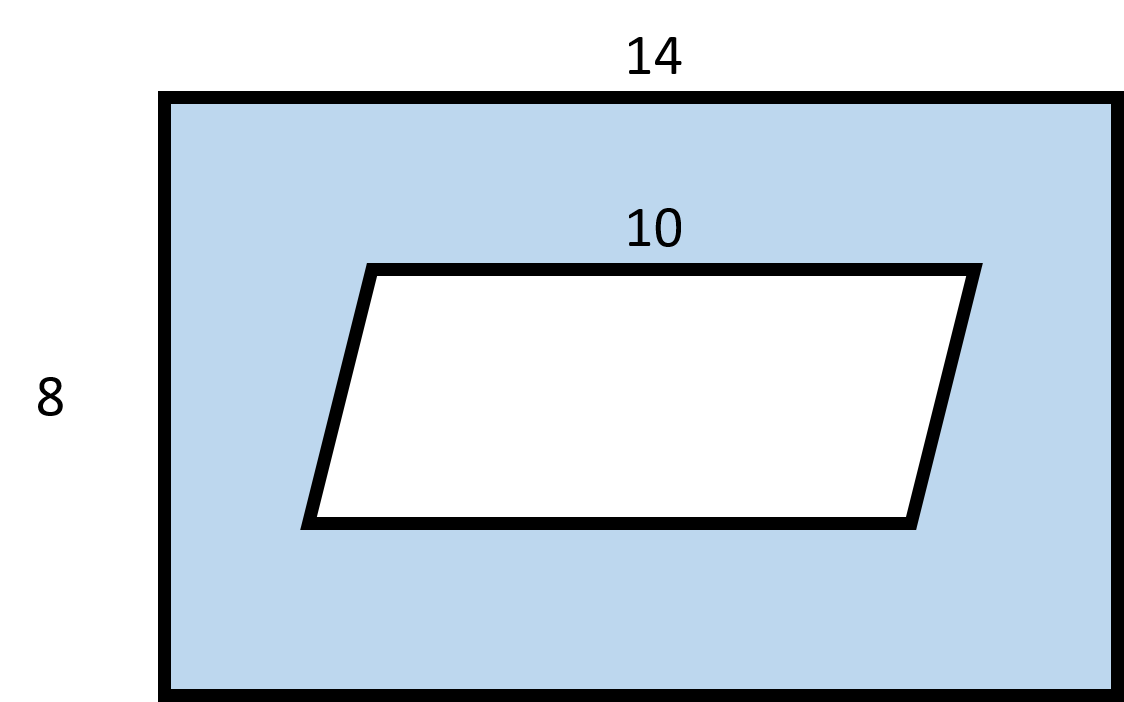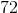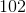Explanation: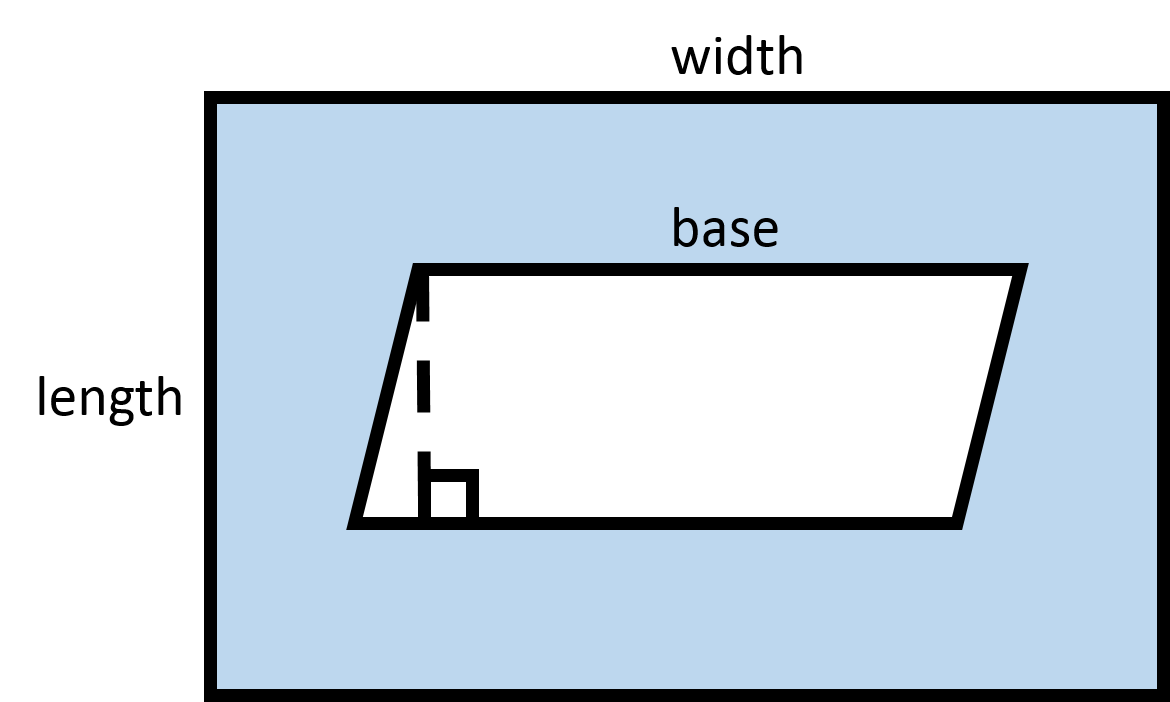In order to find the area of the shaded region, we must first find the areas of the rectangle and parallelogram.

Recall how to find the area of a rectangle: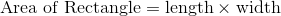Substitute in the given length and height to find the area of the rectangle.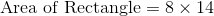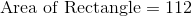Next, find the area of the parallelogram.

Recall how to find the area of a parallelogram: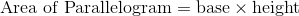We need to find the height of the parallelogram. From the question, we know the following relationship: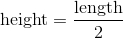Substitute in the length of the rectangle to find the height of the parallelogram.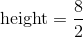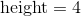Now, substitute in the height and the given length of the base to find the area of the parallelogram.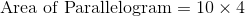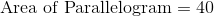Now, we are ready to find the area of the shaded region by subtracting the area of the parallelogram from the area of the rectangle.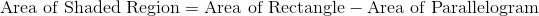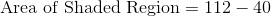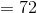1 2 3 4 6 Next →

### All Intermediate Geometry Resources